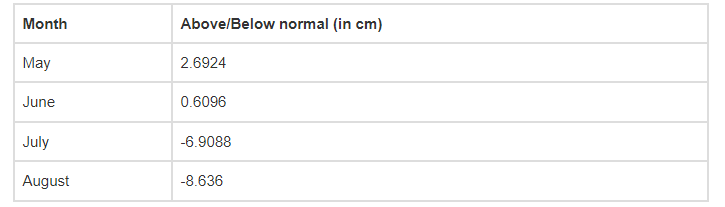# Here is a table which gives the information

Question:

Here is a table which gives the information about the total rainfall for several months compared to the average monthly rains of a town. Write each decimal in the form of rational number p/q.Solution:

(i) May

2.6924 cm = 26924/10000 … [∵by the decimal removing method]

By dividing both numerator and denominator by 4 we get,

= 6731/2500 cm

(ii) June

0.6096 cm = 0.6096/10000 … [∵by the decimal removing method]

By dividing both numerator and denominator by 16 we get,

= 381/625 cm

(iii) July

-6.9088 cm = -69088/10000 … [∵by the decimal removing method]

By dividing both numerator and denominator by 4 we get,

= -4318/625 cm

(iv)August

-8.636 cm = -8636/1000 … [∵by the decimal removing method]

By dividing both numerator and denominator by 4 we get,

= -2159/250 cm